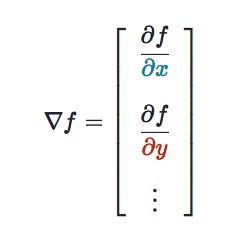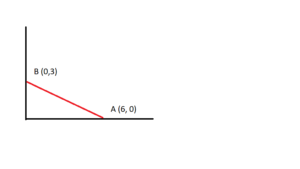The term gradient has at least two meanings in calculus. It usually refers to either:

• The slope of a function. For example, the AS Use of Maths Textbook 2004 mathematics textbook states that “…straight lines have fixed gradients (or slopes)” (p.16). Many older textbooks (like this one from 1914) also tend to use the word gradient to mean slope.
• A specific type of multivariable derivative.

This article is mainly concerned with the gradient as it relates to partial derivatives. If you want an introduction to slopes, see: what is a slope? You can also find some simple examples of finding a gradient (slope) below.

A gradient can refer to the derivative of a function. Although the derivative of a single variable function can be called a gradient, the term is more often used for complicated, multivariable situations where you have multiple inputs and a single output. In those cases, the gradient vector stores all the partial derivative information for every variable.For a function f, the gradient is typically denoted grad f or Δf.

## Finding the Gradient for Multi-Variable Functions

To find the gradient for multi-variable functions, find the partial derivatives for each variable.

Example question: Find Δf for the function
f(x,y) = x2 + y3.

Step 1: Find the partial derivative of f with respect to x. To do this, leave y constant, leaving you to find only the derivative of x2, which is 2x (I used the power rule here). That gives:
2x + y3 = 2xy3.

Step 2: Find the partial derivative of f with respect to y. This time, leave x constant; find just the derivative of y3, which is 3y2. This gives:
x2 + 2y2 = 3x2y2

Step 3: Rewrite your answers from the preceding steps in Δf format, which is just like writing coordinates (x,y):

This is the gradient for the stated function, but sometimes you might want to find it with certain inputs, like grad f(1, 2). To do that, just replace x, y in the partial derivatives with (1, 2).

Note: It doesn’t matter how many variables there are; the procedure is the same. So to find the grad of w = g(x,y,z), take the partial derivative of x, y, and z, respectively. Since g has three variables, Δg has three terms.

## Basic Examples

Often you’re given a graph with a straight-line and asked to find the gradient of the line. You could use the following formula:

G = Change in y-coordinate / Change in x-coordinate
This is sometimes written as
G = Δy / Δx

Let’s take a look at an example of a straight line graph with two given points (A and B).Filling in the coordinates for points A and B:

G = (3-0)/(0-6) = 3/-6 = -1/2

In this example, the gradient is -½.  You could also use the gradient to find the equation of the above line (the equation for a linear function is y = mx + b). The line crosses the y-axis at point B when y = 3.  Therefore, the equation would be y = -½ + 3.

## The Gradient of a Curve

In addition a straight line you may be asked to find the gradient of a curve.  In order to calculate it, you could draw a tangent line to the curve— a line which touches the curve at one point.  You then calculate the gradient of that single line—the tangent.

Example:

Find the gradient of the curve at point (2, 7)As you can probably see on the graph above, the tangent touches the curve around point (1, 3).  You could use the same formula as the formula for a straight line:(change in y)/(change in x)

Plugging the numbers into the formula:

(7-3)/2-1) = 4/1

The gradient of the curve at the point (2, 7) is 4.

## References

 Haighton, J. Haworth, A. (2004). AS Use of Maths. Nelson Thornes.
Godfrey, C. & Sinnons, A. (1914). First steps in the calculus. Cambridge University Press.
Haighton, J. & Haworth, A (2004). AS – Use of Maths – Calculus. Nelson Thornes.